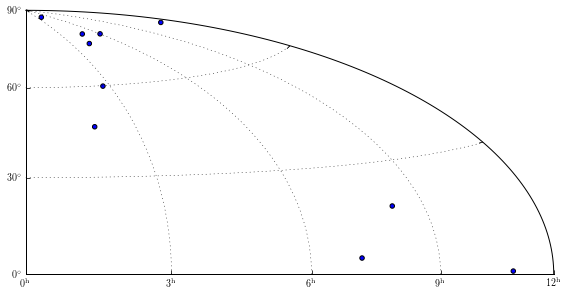In :
import numpy as np
import matplotlib.pyplot as plt

from matplotlib.transforms import Affine2D
from matplotlib.projections import HammerAxes

import mpl_toolkits.axisartist.floating_axes as floating_axes
import  mpl_toolkits.axisartist.angle_helper as angle_helper


In :
def setup_axes(fig, rect):
"""
Sometimes, things like axis_direction need to be adjusted.
"""

tr_scale = Affine2D().scale(np.pi/180., np.pi/180.)

tr = tr_scale + HammerAxes.HammerTransform(resolution=1)

grid_locator1 = angle_helper.LocatorHMS(4)
tick_formatter1 = angle_helper.FormatterHMS()

grid_locator2 = angle_helper.LocatorDMS(3)
tick_formatter2 = angle_helper.FormatterDMS()

ra0, ra1 = 0, 180
dec0 , dec1 = 0, 90
grid_helper = floating_axes.GridHelperCurveLinear(tr,
extremes=(ra0, ra1, dec0, dec1),
grid_locator1=grid_locator1,
grid_locator2=grid_locator2,
tick_formatter1=tick_formatter1,
tick_formatter2=tick_formatter2,
)

ax1 = floating_axes.FloatingSubplot(fig, rect, grid_helper=grid_helper)

# create a parasite axes whose transData in RA, cz
aux_ax = ax1.get_aux_axes(tr)

aux_ax.patch = ax1.patch # for aux_ax to have a clip path as in ax
ax1.patch.zorder=0.9 # but this has a side effect that the patch is
# drawn twice, and possibly over some other
# artists. So, we decrease the zorder a bit to
# prevent this.

return ax1, aux_ax

In :
fig = plt.figure(1, figsize=(8, 5))

ax, aux_ax = setup_axes(fig, 111)

theta = np.random.rand(10)*180. # in degrees